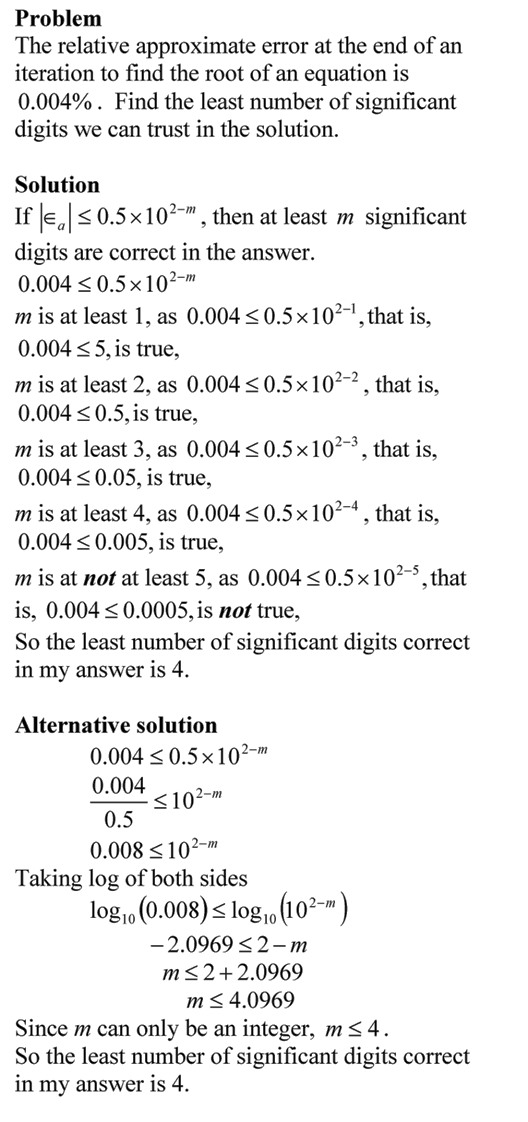## Example: How many significant digits are correct in my answer?

In the previous post I showed you the theorem that tells us how many significant digits are correct in our numerical solution that is iterative in nature.  In this post, we illustrate this with an example.__________________________________________
This post is brought to you by Holistic Numerical Methods: Numerical Methods for the STEM undergraduate at http://numericalmethods.eng.usf.edu, the textbook on Numerical Methods with Applications available from the lulu storefront, the textbook on Introduction to Programming Concepts Using MATLAB, and the YouTube video lectures available at http://numericalmethods.eng.usf.edu/videos.  Subscribe to the blog via a reader or email to stay updated with this blog. Let the information follow you.

Advertisements

## How many significant digits are correct in my answer?

To find how many significant digits are correct in my answer in a numerical method that gives iterative values, one finds the absolute relative approximate  percentage error defined as
|(Current approximation-Previous approximation)/Current approximation|*100
If the absolute relative approximate percentage error is less than or equal to 0.5*10^(2-m), then m significant digits are at least correct in the answer.
For example, if you want

• at least 1 signficant digit to be correct in your answer, your absolute relative approximate error should be less than or equal to 5%
• at least 2 signficant digit to be correct in your answer, your absolute relative approximate error should be less than or equal to 0.5%
• at least 3 signficant digit to be correct in your answer, your absolute relative approximate error should be less than or equal to 0.05%
and so on.

In the next blog, we will illustrate this concept via an example.
___________________________________________
This post is brought to you by Holistic Numerical Methods: Numerical Methods for the STEM undergraduate at http://numericalmethods.eng.usf.edu, the textbook on Numerical Methods with Applications available from the lulu storefront, the textbook on Introduction to Programming Concepts Using MATLAB, and the YouTube video lectures available at http://numericalmethods.eng.usf.edu/videos.  Subscribe to the blog via a reader or email to stay updated with this blog. Let the information follow you.